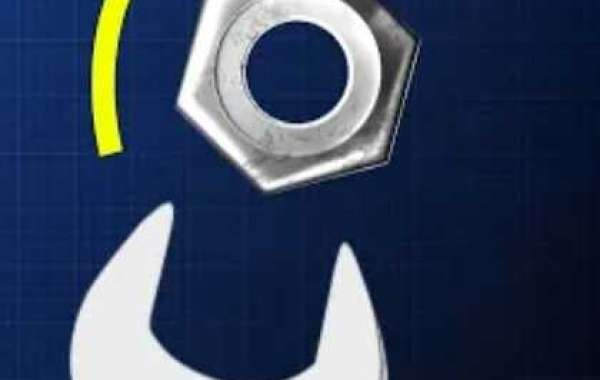### What Is Torque?

• Posted By : Muhammad Nafis Uddin
• 227 views• When we try to open the door of any house,  we apply a rotational force towards a certain direction and that causes the door to be opened. This force is called TORQUE.
• Torque is a force that causes rotational motion of an object about one or more axis that pass through of its center of mass.
• It is defined as the vector peoduct of the position vector and the force vector. It is a vector which perpendicular to both the positon vector and the force vector. As a result,  the torque of a vector is perpendicular to the plane defined by the position vector and the force vector.
• The direction of torque is given by the right-hand thumb rule. To apply the right-hand thumb rule, one have to put his fingers of right hand along the position vector and bend them towards force vector. And the direction of the thumb will give the direction of Torque.
• It is denoted by the greek symbol tau( )